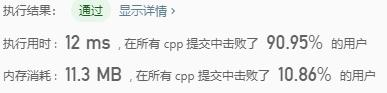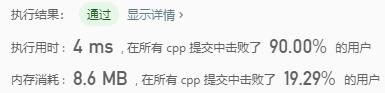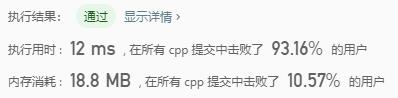# Leetcode 2：两数相加

## 代码实现

ListNode* addTwoNumbers(ListNode* l1, ListNode* l2) { if(l1==NULL) return l2; if(l2==NULL) return l1; ListNode *tmp=new ListNode(-1); ListNode *res=tmp; ListNode *add1=l1; ListNode *add2=l2; int ge=0; int jin=0; while(add1!=NULL || add2!=NULL||jin) { ge=(add1?add1->val:0)+(add2?add2->val:0)+jin; jin=ge/10; ge=ge%10; ListNode *newnode = new ListNode(ge); tmp->next=newnode; tmp=tmp->next; if(add1) add1=add1->next; if(add2) add2=add2->next; } return res->next; }

## 执行用时&内存消耗# Leetcode 3：无重复字符的最长子串

## 代码实现

int lengthOfLongestSubstring(string s) {        int len = s.size();        vector<int> a(256,-1);        int maxlen = 0;        int l = -1;        for(int i=0; i<len; i++){            l = max(l,a[s[i]]);            a[s[i]] = i;            maxlen = max(maxlen,i-l);        }               return maxlen;     }

## 执行用时&占用空间# Leetcode 72：编辑距离

1. 插入一个字符
2. 删除一个字符
3. 替换一个字符

## 示例

horse -> rorse (将 ‘h’ 替换为 ‘r’)
rorse -> rose (删除 ‘r’)
rose -> ros (删除 ‘e’)

## 代码实现

int min3(int a, int b, int c){    return (a < b) ? (a < c ? a : c) : (b < c ? b : c);}int minDistance(string word1, string word2) {    int strlen1 = word1.size();    int strlen2 = word2.size();    vector<vector<int>>dp(strlen1 + 1, vector<int>(strlen2 + 1, 0));    for (int i = 1; i <= strlen1; i++)        dp[i] = i;    for (int i = 1; i <= strlen2; i++)        dp[i] = i;    for (int i = 1; i <= strlen1; i++)    {        for (int j = 1; j <= strlen2; j++)        {            int diff;            if (word1[i - 1] == word2[j - 1])                diff = 0;            else                diff = 1;            dp[i][j] = min3(dp[i - 1][j] + 1, dp[i][j - 1] + 1, dp[i - 1][j - 1] + diff);        }    }    return dp[strlen1][strlen2];}

## 复杂度# Leetcode 75：颜色分类

## 解题思路

1. 若current==0，因为0要排在前面，所以则将start指向的值和current的值交换，然后start++,current++；
2. 若current==1，因为1是排在中间，所以current++，start不变；
3. 若current==2，则将current和end的元素交换，将end–，但是current不变，如果current后移1位，那么current这一位就无法判断了。

## 代码实现

void sortColorsl(vector<int> &nums){ int len = nums.size(); int start = 0, current = 0, end = len - 1; //在current<=end的背景下，然后开始判断current的值 while (current <= end) { //1.若current==0，则将start指向的值和current的值交换，然后start++,current++； if (nums[current] == 0) { swap(nums[current], nums[start]); ++current; ++start; } //3.若current==2，则将current和end的元素交换，将end--，但是current不变。 else if (nums[current] == 2) { swap(nums[current], nums[end]); --end; } //2.若current==1，则current++，start不变； else if (nums[current] == 1) { ++current; } }}

## 复杂度# Leetcode 76：最小覆盖子串

## 代码实现

string minWindow(string s, string t){    int count = { 0 };    for (auto c : t)++count;//统计t中每个字符出现的次数    int len = 0, minlen = s.size();//minlen最大为s的长度    string res;    for (int l = 0, r = 0; r < s.size(); ++r) {        if (--count[s[r]] >= 0)++len;        //上面这句话的意思是：不断移动窗口的right，同时若在s中发现t中有的字符，则len++        //直到len加到和t相同，也就是在s中找到了一个字符串        //下面就是找最优字符串了        while (len == t.size()) {            if (r - l + 1 <= minlen) {                minlen = r - l + 1;                res = s.substr(l, minlen);            }            if (++count[s[l++]] > 0)--len;        }                }    return res;}

## 复杂度